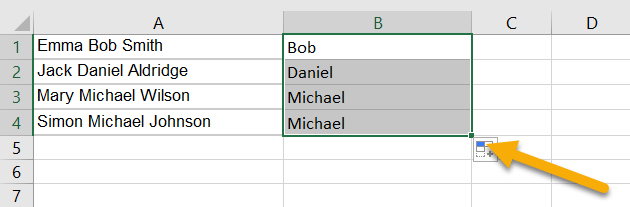# How to☝️ Remove the Middle Name from a Full Name in Excel

To remove the middle name from a full name in Excel, click on the cell where you want the result, go to the Formula bar, enter the formula
=IF(COUNTIF(A1,”* * *”),REPLACE(A1,FIND(” “,A1)+1,FIND(” “,A1,FIND(” “,A1)+1)-FIND(” “,A1),””),””), and hit Enter

Scroll down to find out more about how you can remove the middle name from a full name in just a few seconds without any hassle. This article will cover two methods of how to do this—choose whichever works best for you.

Quick Jump

## Method 1: Using a Custom Formula

One of the easiest ways to remove a person’s middle name is to use a formula. So, let’s take a look at the formula below.

Difficulty: Beginner

Time Estimate: 10 seconds

1. Select the cell where you want the result.
2. Navigate to the Formula bar and enter the formula =IF(COUNTIF(A1,”* * *”),REPLACE(A1,FIND(” “,A1)+1,FIND(” “,A1,FIND(” “,A1)+1)-FIND(” “,A1),””),””), where A1 is the cell with the full name whose middle name you need to remove.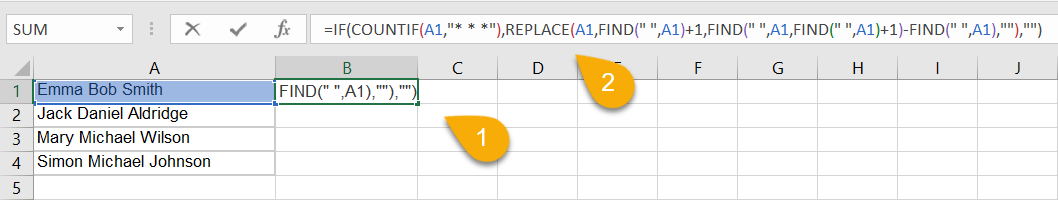1. Press the Enter key on your keyboard.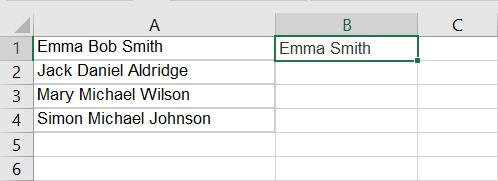Just like that, the name has been rewritten with the middle name taken out.

If you have a dataset and want to apply this formula to the rest of the values in your list, simply do the following:

1. Click and hold the icon in the lower right corner of the cell with the formula and drag downward through the rest of the list.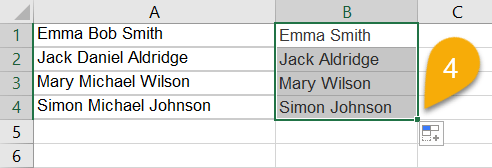As you can see below, the formula has been applied to each name in the list.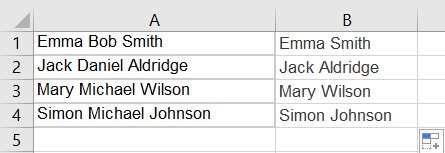## Method 2: Using the Find and Replace Tool

The Find and Replace tool is a powerful search and replace tool for Microsoft Excel that you can use to find and replace both simple and complex patterns of data. Let’s see how it works when it comes to removing middle names!

Difficulty: Beginner

Time Estimate: 10 seconds

1. Select the cells with names that you want to alter.
2. Press the Ctrl + H (Windows) / Cmd + Shift + H (Mac OS) key combination to bring up the Find and Replace dialog box.
3. Add the “ * “ symbol in the Find what row. Don’t forget to put a space before and after the asterisk ( * ) symbol.
4. In the Replace with string, enter a space. This will replace the name with a blank space.
5. Hit the Replace All button.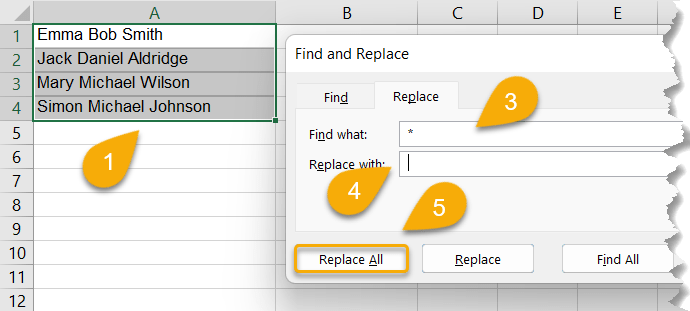1. Confirm your action by clicking OK.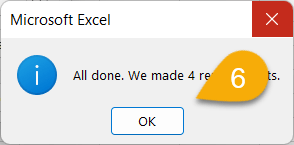Voila! You have successfully removed the middle names from your data.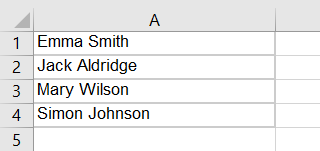## How to Remove the Middle Name in Excel FAQs

### How do I remove the middle name from a name listed as “last, first, middle” in Excel?

To remove a middle name from a name that is written in the form “last, first, middle” in Excel, click on the cell where you want the result, go to the Formula bar, and enter the formula
=LEFT(A1,FIND(” “,A1,FIND(” “,A1)+1)-1). Here, A1 is the cell with the full name.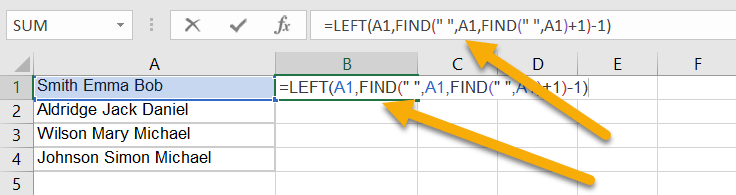Next, click Enter and drag the cell with the formula downward from the bottom right corner icon to apply the formula to the rest of the list.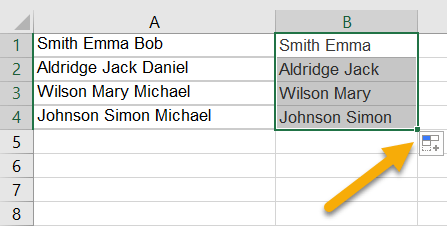### How do I remove initials from names in Excel?

To remove initials from names in Excel, pick the cell where you want the result. Next, in the Formula bar, type the formula =LEFT(A1,FIND(” “,A1))&TRIM(RIGHT(SUBSTITUTE(A1,” “,REPT(” “,99)),99)).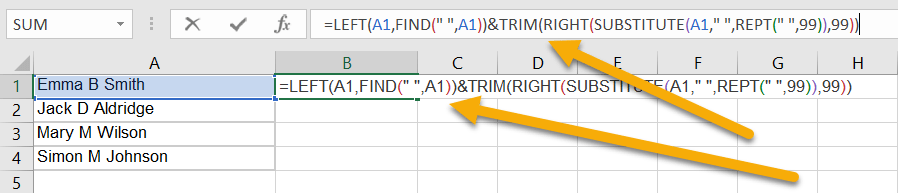Hit Enter and drag the cell with the result downward to copy the formula for the rest of the list.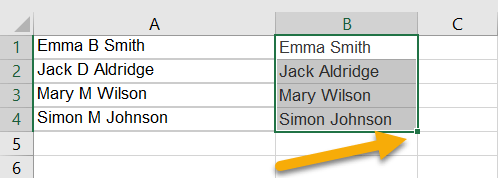### How do I remove the middle initial when only some names have one?

To remove the middle initial when only some names in the list have one, select the blank cell where you want the result, go to the Formula bar, and enter the formula =LEFT(A1,FIND(“}}}”,substitute(a1&” “,” “,”}}}”,2))-1)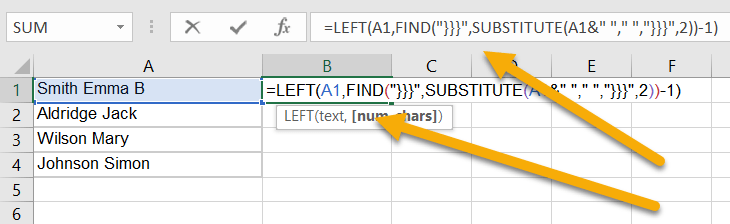Press Enter. Then drag the cell with the result downward to copy the formula for the rest of the names.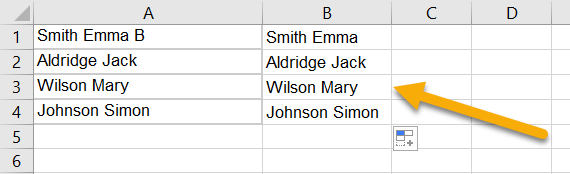### How do I extract middle names in Excel?

To extract middle names in Excel, choose a blank cell, navigate to the Formula bar, and type the formula =MID(A1,SEARCH(” “,A1,1)+1,SEARCH(” “,A1,SEARCH(” “,A1,1)+1)-SEARCH(” “,A1,1)), where A1 is the cell with the full name.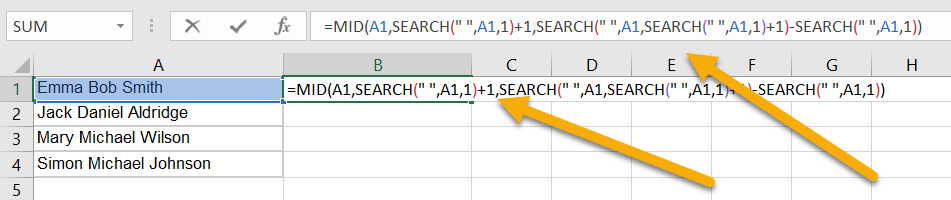Finally, hit the Enter key on your keyboard. Drag the cell downward to copy the formula for the rest of the list.